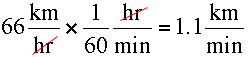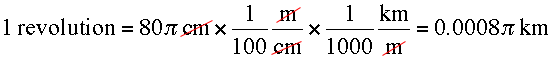SEARCH HOMEMath Central Quandaries & QueriesQuestion from varoon, a student: The wheels of a car are of diameter 80cm each. How many complete revolutions does each wheel make in 10 minutes when the car is travelling at a speed of 66kms per hour?Varoon,

66 km per hour isThe car travels at this speed for 10 minutes and hence it travels 11 km.

The diameter of the wheel is 80 cm so its circumference measures 80 π cm. thusSo how many revolutions are there in 11 km?

PennyMath Central is supported by the University of Regina and The Pacific Institute for the Mathematical Sciences.